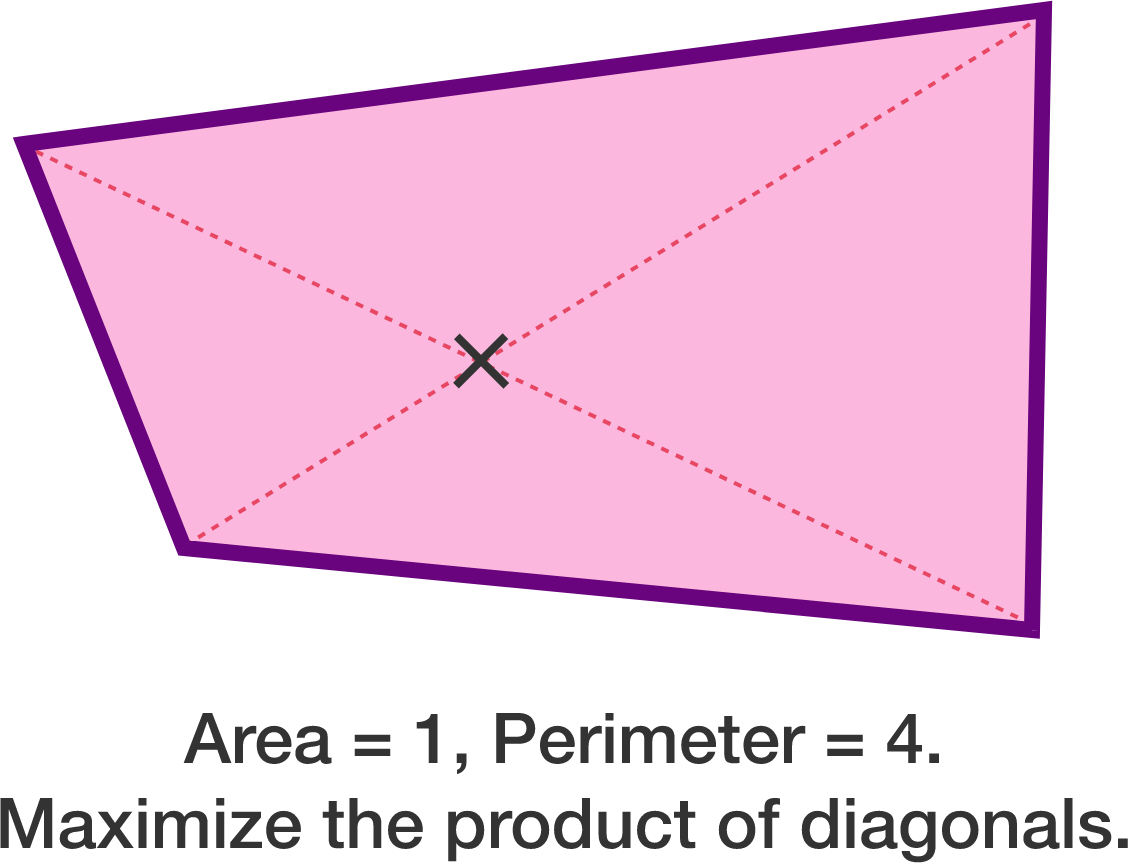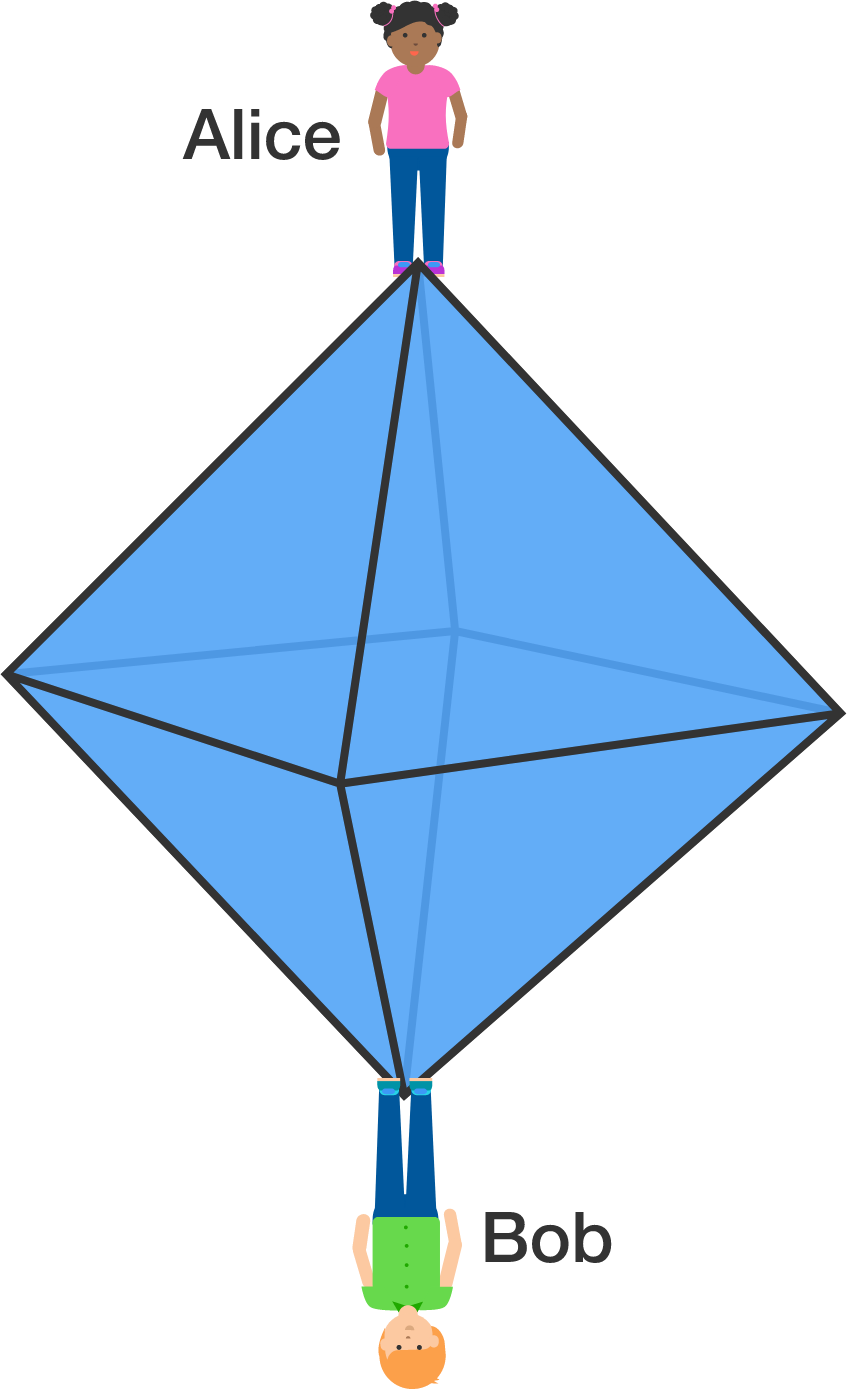# Problems of the Week

Contribute a problem

I have a quadrilateral with perimeter 4 and area 1.

Find the maximum value of the product of its diagonal lengths to 2 decimal places.What is the minimum value of $n$ such that there exist integers $a_1, a_2, \ldots, a_n$ that satisfy

$a_1^5+a_2^5+\cdots+a_n^5=28?$Alice and Bob stand on opposite vertices of a regular octahedron. At the beginning of every minute,

• each chooses an adjacent vertex uniformly at random and moves towards it;
• each moves at a constant rate of 1 edge per minute;
• they will stop when they meet up.

The expected value of the number of minutes until they meet up is equal to $\frac{p}{q}$, where $p$ and $q$ are coprime positive integers.

Find the value of $p + q.$


Note: It is possible that they would meet at a vertex or at the midpoint of an edge.

Evaluate $\lim_{n \to \infty} \displaystyle \int_0^1 \int_0^1 \ldots \int_0^1 \dfrac{n}{x_1+x_2+\dots+x_n}\, dx_1 dx_2 \ldots dx_n.$

Clarification: In the answer options, $e \, (\approx 2.71828)$ is the Euler's number.

###### Note: This problem is a generalization of my previous problem.

You have recently bought the Random Number Generator 4000 from your local hardware store. This remarkable machine outputs numbers randomly and uniformly in the range $[0, 1]$. You decide to create a list of these numbers and find the total sum.

What is the expected number of times the machine should operate to generate a number before the total sum is at least 1?